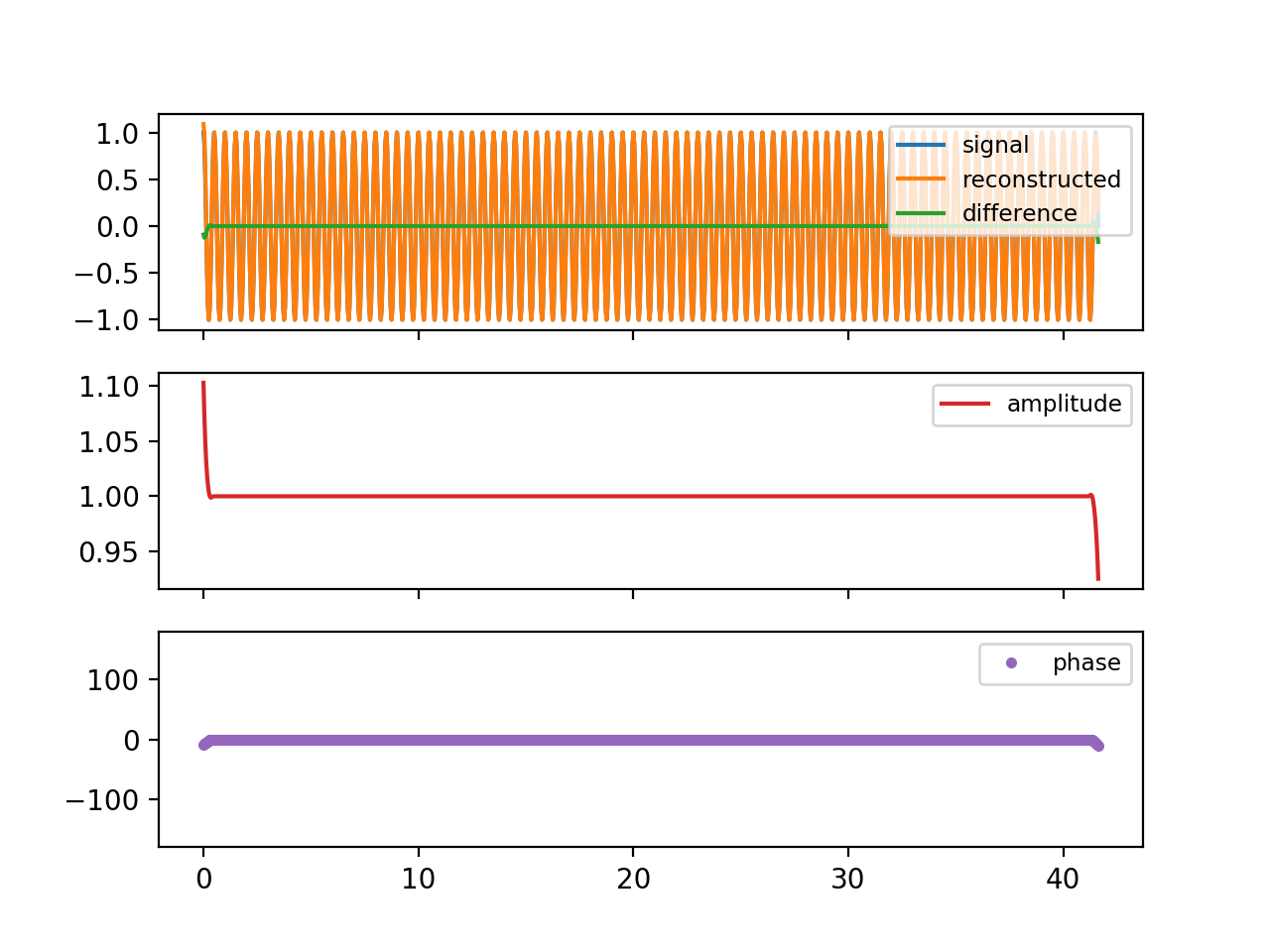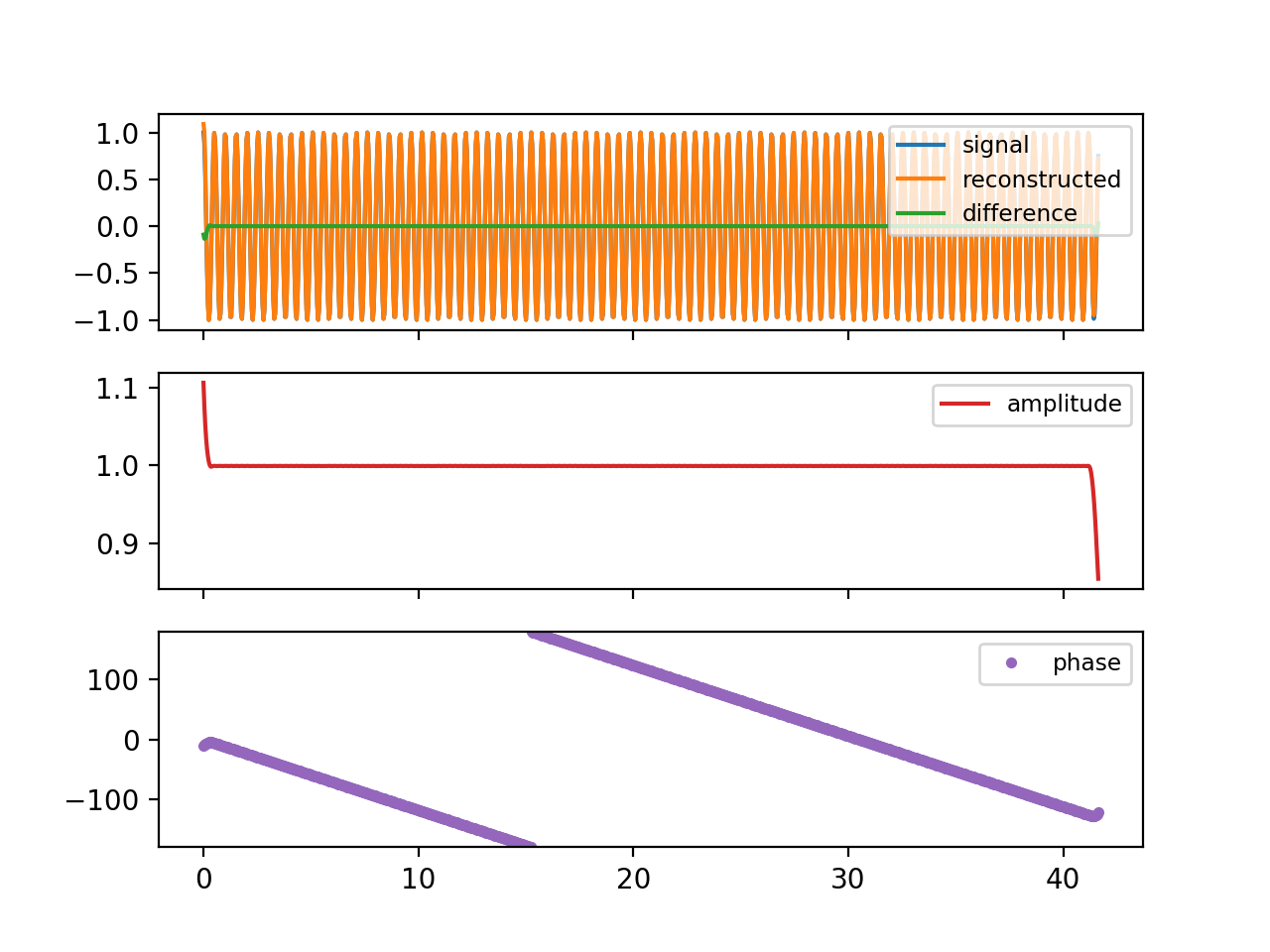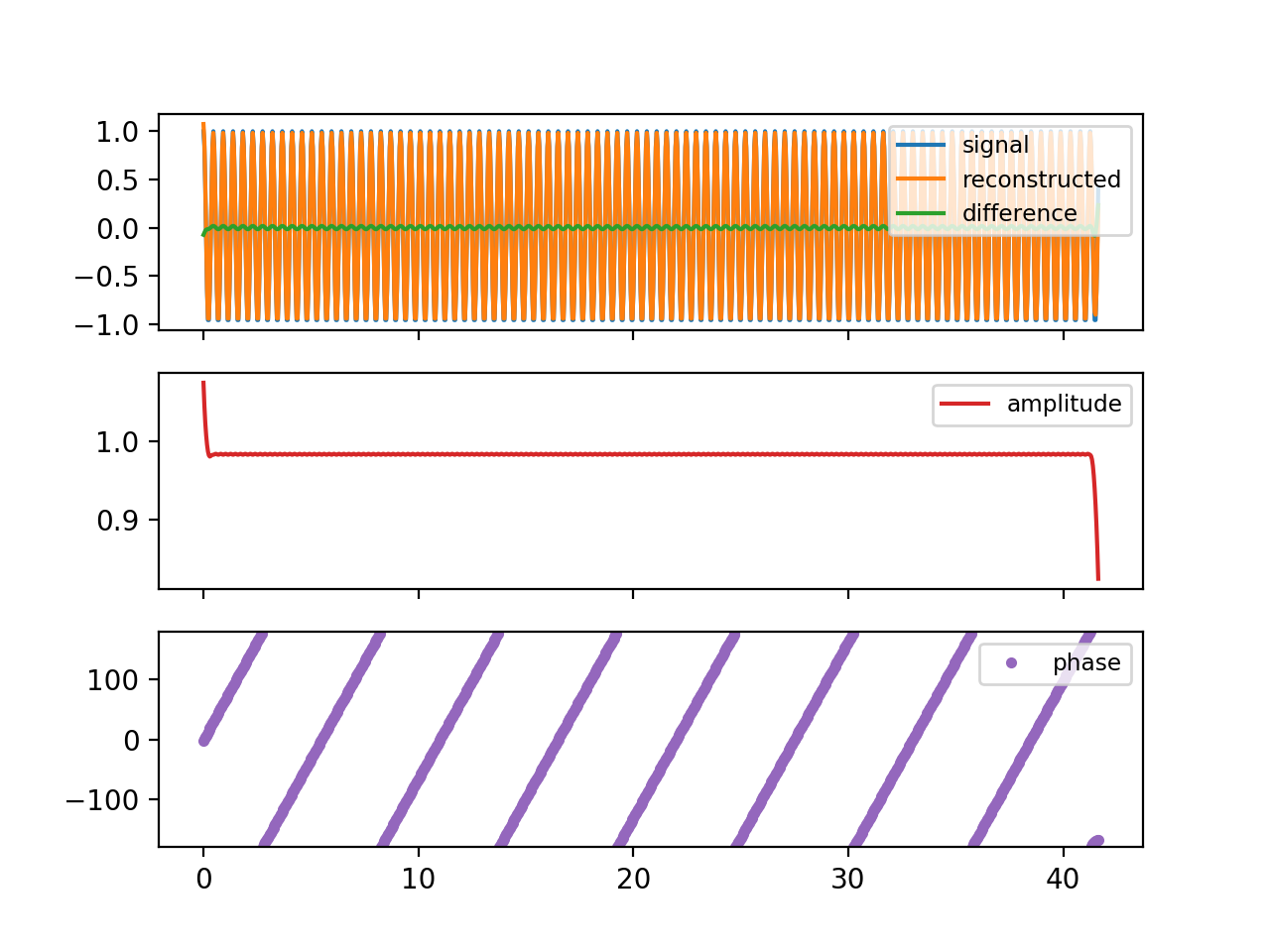# Complex demodulation¶

The concepts of modulation and demodulation arise naturally in the context of transmitting information via radio. There are two basic kinds of modulation used to transmit audio via radio frequency signals: amplitude modulation (AM) and frequency modulation (FM). In both cases the fundamental radio frequency of the signal is the "carrier frequency", which would be a perfect sinusoid in the case of no modulation (no audio signal). For AM, the carrier is multiplied by the signal in the audio frequency band (20 Hz to 20 kHz). For FM, the frequency of the carrier is shifted up and down with the audio signal waveform. In either case, the job of the receiver is to isolate the modulated signal from all the other radio-frequency energy, and extract the original audio signal from it--which is called "demodulation", of course. The way this is done in an AM receiver is essentially identical to the data analysis technique called "complex demodulation", where the "complex" has nothing to do with complexity. It is merely a reference to the use of complex exponentials, which makes the mathematical description and the programming code more concise. The technique is really very simple, and can be implemented with or without the use of complex numbers.

Here's the recipe:

• multiply your time series by a complex exponential at the frequency of interest
• apply a low-pass filter to the product
• look at the amplitude and phase of the resulting complex time series

As this recipe indicates, the purpose of complex demodulation is to isolate a fairly narrow frequency band and describe it as a sinusoid that can be modulated in amplitude (which we see in the amplitude part of the complex demodulation output) and in frequency (which we see in the rate of change of phase with time). For example, it can be used to isolate the spring-neap cycle of the semidiurnal tide--an example of amplitude modulation resulting from the superposition of the M2 and S2 tidal constituents.

Here is the mathematical version of the recipe. Recall that the time series can be represented as a sum of sinusoids via its Fourier Transform:

$$x(t_n) = \frac{1}{N} \sum_{k=0}^{N-1} A_k \exp(i 2 \pi f_k t_n).$$

Then

$$x(t_n) \exp(-i 2 \pi f t_n) = \frac{1}{N} \sum_{k=0}^{N-1} A_k \exp(i 2 \pi (f_k-f) t_n)$$

so that each component at $f_k$ has been shifted down to frequency $f_k - f$. For example, if $f_k$ and $f$ are both 1 cycle per year, then the energy at 1 cycle per year is shifted to zero-frequency--that is, it is appears in the mean. Using a low-pass filter will isolate the energy that is near frequency $f$. The amplitude of the low-passed complex product will vary slowly with time, as the amplitude of the energy near $f$ varies. The phase will vary slowly in time, depending on how the frequency content of that energy compares to $f$.

Recall a central concept from Fourier analysis: the uncertainty principle stating that there is always a tradeoff between localization in time and localization in frequency. A narrow bandwidth signal has a modulation with a large time scale, and a pulse-like signal has a broad bandwidth. This concept applies to complex demodulation (and to its relative, wavelet analysis). It is just as inescapable as the uncertainty principle in quantum physics.

## A real-valued time series¶

Recall that if $x(t_n)$ is real rather than complex, the Fourier coefficients for the frequencies above Nyquist, or equivalently for the negative frequencies, are the complex conjugates of their counterparts in the first half of the transform:

$$A_{-k} = A_k^*$$

Then

$$x(t_n) = \frac{1}{N} \left\{ A_0 + A_{N/2}\exp(i 2 \pi f_{N/2} t_n) + \sum_{k=1}^{N/2-1} [A_k \exp(i 2 \pi f_k t_n) +A_k^* \exp(-i 2 \pi f_k t_n)]\right\}$$

and

$$x(t_n) \exp(-i 2 \pi f t_n) = \frac{1}{N} \left\{ A_0 \exp(-i 2 \pi f t_n) + A_{N/2}\exp(i 2 \pi (f_{N/2}-f) t_n)\\ + \sum_{k=1}^{N/2-1} [A_k \exp(i 2 \pi (f_k -f) t_n) +A_k^* \exp(-i 2 \pi (f_k+f) t_n)]\right\}$$

.

Notice that we now have contributions at all sum and difference frequencies between the demodulation frequency, $f$, and each of the positive Fourier frequencies through the Nyquist. We use a low-pass filter to keep only the energy from the difference between $f$ and nearby Fourier frequencies.

## A little experimentation¶

In :
%matplotlib notebook

import numpy as np
import matplotlib.pyplot as plt
from pycurrents.system import Bunch


We need a set of filter weights, so we will use a Blackman.

In :
def bl_filt(y, half_width):
"""
Simple Blackman filter.

The end effects are handled by calculating the weighted
average of however many points are available, rather than
"""
nf = half_width * 2 + 1
x = np.linspace(-1, 1, nf, endpoint=True)
x = x[1:-1]   # chop off the useless endpoints with zero weight
w = 0.42 + 0.5 * np.cos(x * np.pi) + 0.08 * np.cos(x * 2 * np.pi)
ytop = np.convolve(y, w, mode='same')
ybot = np.convolve(np.ones_like(y), w, mode='same')

return ytop / ybot


We also need test data. The function below could be modified to permit input of different amplitudes and phases for the periodic components, and even different spectral characteristics of the noise; but the simple version here is adequate for present purposes.

In :
def test_data(periods, noise=0, rotary=False, npts=1000, dt=1.0/24):
"""
Generate a simple time series for testing complex demodulation.

*periods* is a sequence with the periods of one or more
harmonics that will be added to make the test signal.
They can be positive or negative.
*noise* is the amplitude of independent Gaussian noise.
*rotary* is Boolean; if True, the test signal is complex.
*npts* is the length of the series.
*dt* is the time interval (default is 1.0/24)

Returns t, x: ndarrays with the test times and test data values.

Note: the default of dt = 1/24 corresponds to hourly values in
units of days, so a period of 12.42/24 would give the M2 frequency.
"""

t = np.arange(npts, dtype=float) * dt

if rotary:
x = noise * (np.random.randn(npts) + 1j * np.random.randn(npts))
else:
x = noise * np.random.randn(npts)

for p in periods:
if rotary:
x += np.exp(2j * np.pi * t / p)
else:
x += np.cos(2 * np.pi * t / p)

return t, x



Now we will provide a basic complex demodulation implementation for 1-D inputs. Notice that in general it needs to be called twice for complex inputs because it yields only one rotary component at a time. With a few more lines of code it could be modified to yield both components in a single call.

In :
def complex_demod(t, x, central_period, hwidth = 2):
"""
Complex demodulation of a real or complex series, *x*
of samples at times *t*, assumed to be uniformly spaced.

*central_period* is the period of the central frequency
for the demodulation.  It should be positive for real
signals. For complex signals, a positive value will
return the CCW rotary component, and a negative value
will return the CW component (negative frequency).
Period is in the same time units as are used for *t*.

*hwidth* is the Blackman filter half-width in units of the
*central_period*.  For example, the default value of 2
makes the Blackman half-width equal to twice the
central period.

Returns a Bunch; look at the code to see what it contains.
"""

rotary = x.dtype.kind == 'c'  # complex input

# Make the complex exponential for demodulation:
c = np.exp(-1j * 2 * np.pi * t / central_period)

product = x * c

# filter half-width number of points
dt = t - t
hwpts = int(round(hwidth * abs(central_period) / dt))

demod = bl_filt(product, hwpts)
if not rotary:
# The factor of 2 below comes from fact that the
# mean value of a squared unit sinusoid is 0.5.
demod *= 2

reconstructed = (demod * np.conj(c))
if not rotary:
reconstructed = reconstructed.real

if np.sign(central_period) < 0:
demod = np.conj(demod)
# This is to make the phase increase in time
# for both positive and negative demod frequency
# when the frequency of the signal exceeds the
# frequency of the demodulation.

return Bunch(t=t,
signal=x,
hwpts=hwpts,
demod=demod,
reconstructed=reconstructed)


Make a plotter for our demod output.

In :
def plot_demod(dm):
fig, axs = plt.subplots(3, sharex=True)
resid = dm.signal - dm.reconstructed
if dm.signal.dtype.kind == 'c':
axs.plot(dm.t, dm.signal.real, label='signal.real')
axs.plot(dm.t, dm.signal.imag, label='signal.imag')
axs.plot(dm.t, resid.real, label='difference real')
axs.plot(dm.t, resid.imag, label='difference imag')
else:
axs.plot(dm.t, dm.signal, label='signal')
axs.plot(dm.t, dm.reconstructed, label='reconstructed')
axs.plot(dm.t, dm.signal - dm.reconstructed, label='difference')

axs.legend(loc='upper right', fontsize='small')

axs.plot(dm.t, np.abs(dm.demod), label='amplitude', color='C3')
axs.legend(loc='upper right', fontsize='small')

axs.plot(dm.t, np.angle(dm.demod, deg=True), '.', label='phase',
color='C4')
axs.set_ylim(-180, 180)
axs.legend(loc='upper right', fontsize='small')

for ax in axs:
ax.locator_params(axis='y', nbins=5)
return fig, axs


Almost ready. For convenience, make a single function to generate the test data, run the complex demodulation on it, and plot the result.

In :
def test_demod(periods, central_period,
noise=0,
rotary=False,
hwidth = 1,
npts=1000,
dt=1.0/24):

t, x = test_data(periods, noise=noise, rotary=rotary,
npts=npts, dt=dt)
dm = complex_demod(t, x, central_period, hwidth=hwidth)
fig, axs = plot_demod(dm)
return fig, axs, dm


### Single harmonic at the demodulation frequency¶

In :
test_demod([12.0/24], 12.0/24);### Single harmonic at a slightly lower frequency¶

In :
test_demod([12.2/24], 12.0/24);Notice that the amplitude is now very slightly less than one, and the phase is going backwards with time.

### Single harmonic at a slightly higher frequency¶

In :
test_demod([11.0/24], 12.0/24);Again the amplitude is slightly less than one, and now the phase is increasing with time.

### Two sinusoids bracketing the demodulation frequency¶

In :
test_demod([11.5/24, 12.5/24], 12.0/24);